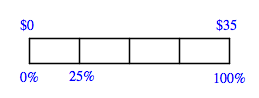### Home > MC1 > Chapter 10 > Lesson 10.1.3 > Problem10-38

10-38.

Multiple Choice: A shirt that normally costs $35$ is on sale for $25\%$ off the original price. What is the sale price? Show how you know.

1. $26.25$

1. $8.75$

1. $43.75$

1. $28.75$

Use the percent ruler below to find the equivalent of $25\%$ of $35$.Once you find the value of the $25\%$ discount, remember that you should subtract that discount from the original price in order to find the new sale price.

The correct price is (a) $26.25$. Did you remember to show how you know?